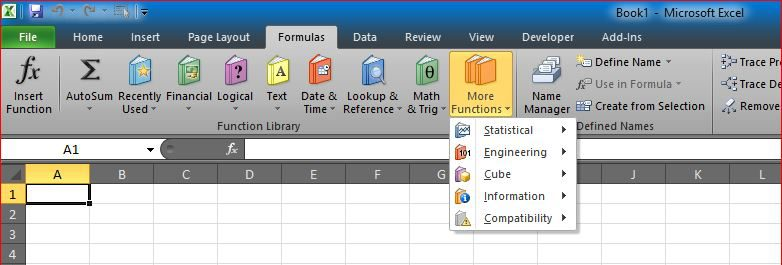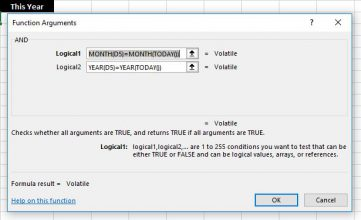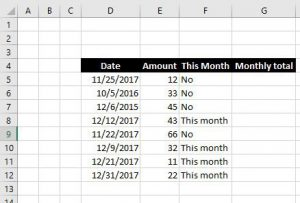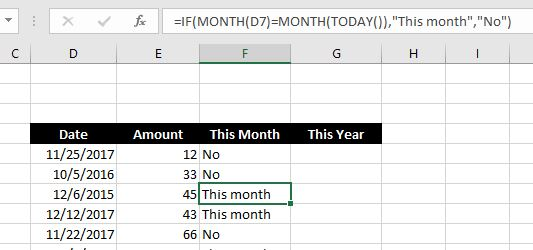# IF with AND and IF with ORThe powerful function If can already do a lot of tricks, but we can for sure do more when we use it with AND or OR functions.

Also, once we understand that, we can use the power of combining AND and OR inside the Logical test of IF, to make it even smarter

Once good example is when we need to see if a certain date is within the same month as this month like below…If you use simple IF with MONTH (one condition)…

`=IF(Month(F2)=Month(Today()),"This Month","")`

You will get “This Month” even if it was not same yearWhich we do not want for sure

So, we can use AND function to make sure both conditions are met at the same time, at that time only, the test will get a TRUE output
``` =IF(AND(MONTH(D5)=MONTH(TODAY()),YEAR(D5)=YEAR(TODAY())),"This month","No")```

AND() function can combine 256 conditions, if all TRUE, it will return a TRUE, while OR() only one of these conditions may return TRUE to result in TRUE

Let me know if that helped in anyway, or not